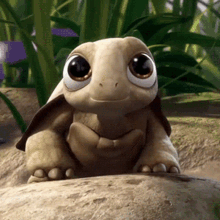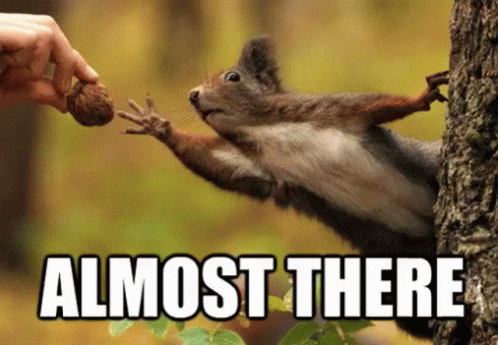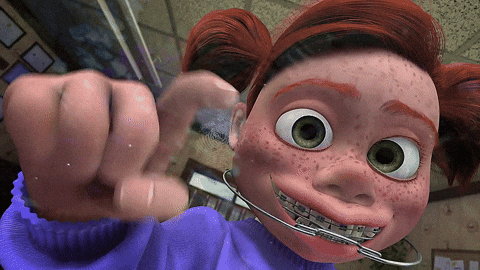# Drawing Shapes with Turtle

## Make a shape drawing program with Python Turtle!@JakeGerber

I like to draw, you like to draw, so let's create a drawing program with shapes such as circles, squares, and triangles! It will turn out really cool.Final demo and code

## Setting up

We're going to use Repl.it, a free, online coding editor, to create the project. Get started by visiting repl.it/languages/python3.Once your repl spins up, let's do this!### Importing Libraries

Let's begin by importing the `turtle` and `math` libraries. At the top of the `main.py` file, add:

``````import turtle
import math
``````

We will be drawing to the screen using the turtle library! `turtle` allows us to draw to the screen like a whiteboard. We can go to specific spots on the screen, draw lines and dots, create filled in shapes, change the drawing color, and more. You'll get to see it in action soon!

The `math` library just provides an easy way to do math in python. We'll use it to make some calculations in a bit.

### Creating Variables

Next, let's create some initial variables:

``````squareWidth = 20
triangleLength = 20
``````

We are creating variables for the width of the square, radius of the circle, and length of the triangle. They will be used in the drawing process later.

Under those variables, add these variables:

``````itemNum = 0
maxItem = 3
``````

The amount of shapes we have will be under `maxItem` and `itemNum` is where we will start (the index in the array). This will make more sense when we draw the items later on.

``````colorNum = 0
colors = ["red", "green", "blue", "yellow", "orange", "purple", "grey", "black"]
``````

The variable `colorNum` is the index we are starting at with the array of "colors". We will handle swtiching between colors later on.

### Initializing The Turtle

Next, let's initialize the turtle.After everything you wrote, at the bottom of the file, add:

``````turtle.hideturtle()
turtle.speed(0)
turtle.up()
``````

Here, we're:

• hiding the turtle cursor, which shows up by default
• setting the speed to 0 so it will draw instantly
• making the turtle's pen go up so it will not draw while moving

## Drawing the Shapes

I know you're eager to get into the meat of the progran, so let's do it.

### Drawing the Circle

We are going to create the circle function. Look at the "o". I know it's a pretty cool circle.At the bottom of the file, add:

``````def circle(x, y):
turtle.begin_fill()
turtle.end_fill()
``````
• First, we define the function, which passes in x and y coordinates as parameters
• Inside the function, we add `begin_fill()` and `end_fill()` statements. Anytime you draw with turtle, you need to wrap your drawing in between these two statements.

In between those two statements, add:

``````def circle(x, y):
turtle.begin_fill()
turtle.end_fill()
``````
• First, we go to the location of where we will draw. It is offset so it'll be centered at the mouse point.
• Then, we draw the circle with `turtle.circle()`!

### Drawing the Square

Next, let's create the square function.Under the `circle()` function, add:

``````def square(x, y):
turtle.begin_fill()
turtle.goto(x-(squareWidth/2), y-(squareWidth/2))
turtle.end_fill()
``````

This look similar to the `circle()` function, except the `goto` statement is optimized for a square, and then we set the direction of the turtle to face right.

Next, just before the `end_fill()` statement but after everything else, add:

``````def square(x, y):
turtle.begin_fill()
turtle.goto(x-(squareWidth/2), y-(squareWidth/2))

for x in range(4):
turtle.forward(20)
turtle.left(90)
turtle.end_fill()
``````

Here, we're:

• Running a for loop 4 times, to account for each side of a square
• Moving the turtle along the square, so that it draws the square.

### Drawing the Triangle

Next, let's create the triangle function.After the `square()` function, add:

``````def triangle(x, y):
turtle.goto(x-(triangleLength/2), y-((triangleLength *math.sqrt(3))/6))
turtle.begin_fill()
turtle.end_fill()
``````

Again, similar to the previous functions, except the `goto()` statement optimizes for a triangle.

Next, in between the `begin_fill()` and `end_fill()` statements, add:

``````def triangle(x, y):
turtle.goto(x-(triangleLength/2), y-((triangleLength *math.sqrt(3))/6))

turtle.begin_fill()
for x in range(3):
turtle.forward(triangleLength)
turtle.left(120)
turtle.end_fill()

``````

Just like how we drew the square, we're drawing the 3 sides of the triangle.

## Finishing Up the ShapesWe are done making the shapes, but that's useless if we can't switch between them! Let's add that.

### Switching Between Shapes

At the bottom of the Python file, after every function you wrote, add:

``````def switchShape(x, y):
global itemNum
global maxItem
``````
• First, we create a function called `switchShape()`, which takes in x and y coordinates as parameters.
• Then, we use the `global` keyword to be able to modify the `itemNum` and `maxItem` global variables we definined at the beginning of this workshop inside this function.

Next, continue the function with the following code:

``````def switchShape(x, y):
global itemNum
global maxItem

itemNum += 1

if (itemNum >= maxItem):
itemNum = 0
``````

Here, we're just incrementing the `itemNum` variable by 1 and resetting it to 0 if it's greater than the `maxItem`.

### Drawing Shapes

Next, let's add a function for drawing shapes. At the bottom of the file, add:

``````def drawItem(x, y):
global itemNum
if (itemNum == 0):
circle(x, y)
elif (itemNum == 1):
square(x, y)
elif (itemNum == 2):
triangle(x, y)
else:
print(itemNum)
``````
• First, we use the `global` keyword again to access the `itemNum` variable.
• If the variable is 0, we draw a circle.
• If it's 1, we draw a square.
• If it's 2, we draw a triangle.
• Otherwise, we print the number to the console since we don't have a shape for it.

### Switching Between Colors

Next, let's add a function to switch between colors.At the bottom of the file, add:

``````def switchColor(x, y):
global colorNum
global colors

colorNum += 1

if (colorNum >= len(colors)):
colorNum = 0

turtle.color(colors[colorNum])
``````
• First, we access the global `colorNum` and `colors` variables.
• Then, we increment the `colorNum` by 1.
• If `colorNum` is greater than the number of items in the `colors` array, we reset it to 0
• Then, we set the turtle's drawing color to the color at the index of the current `colorNum` in the `colors` array.

## Clicking On ScreenWe're almost done! Now, we want to add some code that will handle the user clicking on the screen to draw something. At the bottom of the file, after every function, add:

``````turtle.onscreenclick(drawItem, 1)
turtle.onscreenclick(switchColor, 2)
turtle.onscreenclick(switchShape, 3)
``````
• We call the `turtle.onscreenclick()` function, which is run when it detects that the screen has been clicked.
• If the user clicks the left mouse button (1), we draw the current shape.
• If the user clicks on the middle button (2), we switch the color.
• If the user clicks on the right button (3), we switch the shape.

## Alternative Controls

In case your middle mouse button and right click do not work, let's make some alternative keyboard controls. At the bottom of the file, add:

``````def alternativeControlColor():
canvas = turtle.getcanvas()
x, y = canvas.winfo_pointerx(), canvas.winfo_pointery()
switchColor(x, y)

def alternativeControlShape():
canvas = turtle.getcanvas()
x, y = canvas.winfo_pointerx(), canvas.winfo_pointery()
switchShape(x, y)
``````

These functions get the current mouse position and call their respective function of switching the shape or size.

Next, let turtle know of these alternate controls.

``````turtle.onkey(alternativeControlColor, "s")
turtle.onkey(alternativeControlShape, "d")
``````

Similar to the `onscreenclick()` function, the `onkey` function will automatically run when turtle detects that the user pressed a key. We link the color control to `s` and the shape control to `d`.

``````turtle.listen()
turtle.mainloop()
``````

These two lines make our turtle program run.

## You're done!

You are done! Congrats!Final source code:
``````import turtle
import math

squareWidth = 20
triangleLength = 20

itemNum = 0
maxItem = 3

colorNum = 0
colors = ["red", "green", "blue", "yellow", "orange", "purple", "grey", "black"]

turtle.hideturtle()
turtle.speed(0)
turtle.up()

def drawItem(x, y):
global itemNum
if (itemNum == 0):
circle(x, y)
elif (itemNum == 1):
square(x, y)
elif (itemNum == 2):
triangle(x, y)
else:
print(itemNum)

def circle(x, y):
turtle.begin_fill()
turtle.end_fill()

def square(x, y):
turtle.begin_fill()
turtle.goto(x-(squareWidth/2), y-(squareWidth/2))
for x in range(4):
turtle.forward(20)
turtle.left(90)
turtle.end_fill()

def triangle(x, y):
turtle.goto(x-(triangleLength/2), y-((triangleLength *math.sqrt(3))/6))

turtle.begin_fill()
for x in range(3):
turtle.forward(triangleLength)
turtle.left(120)
turtle.end_fill()

def switchColor(x, y):
global colorNum
global colors

colorNum += 1

if (colorNum >= len(colors)):
colorNum = 0

turtle.color(colors[colorNum])

def switchShape(x, y):
global itemNum
global maxItem

itemNum += 1

if (itemNum >= maxItem):
itemNum = 0

turtle.onscreenclick(drawItem, 1)
turtle.onscreenclick(switchColor, 2)
turtle.onscreenclick(switchShape, 3)

def alternativeControlColor():
canvas = turtle.getcanvas()
x, y = canvas.winfo_pointerx(), canvas.winfo_pointery()
switchColor(x, y)

def alternativeControlShape():
canvas = turtle.getcanvas()
x, y = canvas.winfo_pointerx(), canvas.winfo_pointery()
switchShape(x, y)

turtle.onkey(alternativeControlColor, "s")
turtle.onkey(alternativeControlShape, "d")

turtle.listen()
turtle.mainloop()
``````

## Hacking

The fun doesn't stop here! Here are some things you can do to take this project further: